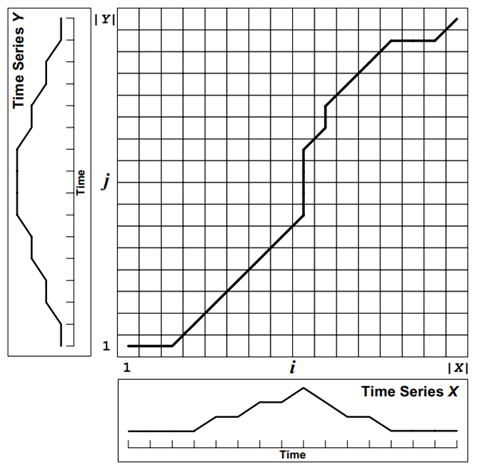# 机器学习之分段线性回归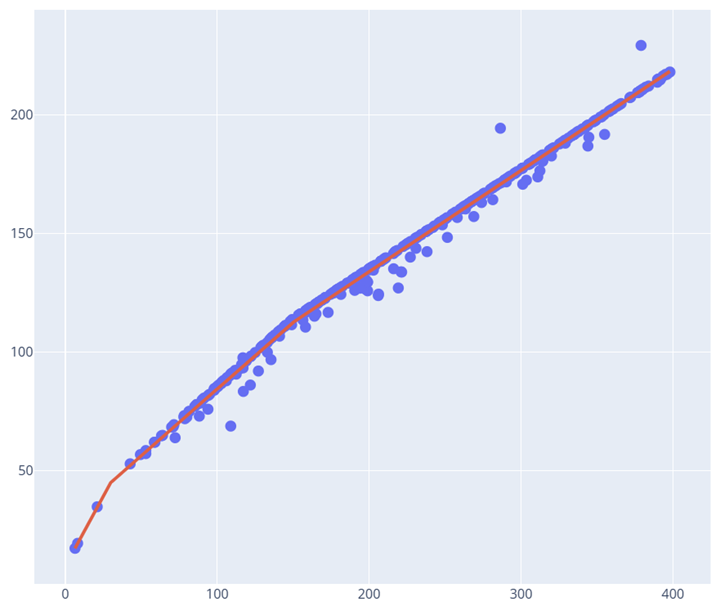## 方法一： 使用numpy.piecewise分段拟合

numpy.piecewise是一个用于创建分段函数的函数，它可以根据指定的条件对输入数组进行分类，并对分类后的每个部分应用不同的操作。下面是 numpy.piecewise 函数的一般语法：

numpy.piecewise(x, condlist, funclist, *args, **kw)

• x：输入数组，可以是任意形状的数组。
• condlist：条件列表，是一个长度为 nnn 的列表，其中包含特定条件下的布尔表达式（或值）。当满足第 kkk 个条件时，piecewise 函数会使用第 kkk 个函数进行操作。
• funclist：函数列表，是一个长度为 nnn 的列表，其中包含了要执行的操作。当满足第 kkk 个条件时，piecewise 函数会使用第 kkk 个函数进行操作。
• *args 和 **kw：可选参数，用于传递给被调用的函数。

import numpy as np

x = np.array([1.5, 2.5, 3.5, 4.5])
y = np.piecewise(x, [x < 2, (x >= 2) & (x < 4), x >= 4], [lambda x: x**2, lambda x: x**3, lambda x: x**4])

print(y) # [ 2.25   15.625  42.875  91.125]


numpy.piecewise进行线性回归代码示例：

from scipy import optimize
import matplotlib.pyplot as plt
import numpy as np

x = np.array([1, 2, 3, 4, 5, 6, 7, 8, 9, 10 ,11, 12, 13, 14, 15], dtype=float)
y = np.array([5, 7, 9, 11, 13, 15, 28.92, 42.81, 56.7, 70.59, 84.47, 98.36, 112.25, 126.14, 140.03])

def piecewise_linear(x, x0, y0, k1, k2):
return np.piecewise(x, [x < x0, x >= x0], [lambda x:k1*x + y0-k1*x0, lambda x:k2*x + y0-k2*x0])

p , e = optimize.curve_fit(piecewise_linear, x, y)
xd = np.linspace(0, 15, 100)
plt.plot(x, y, "o")
plt.plot(xd, piecewise_linear(xd, *p))


from scipy import optimize

def piecewise_linear(x, x0, x1, b, k1, k2, k3):
condlist = [x < x0, (x >= x0) & (x < x1), x >= x1]
funclist = [lambda x: k1*x + b, lambda x: k1*x + b + k2*(x-x0), lambda x: k1*x + b + k2*(x-x0) + k3*(x - x1)]
return np.piecewise(x, condlist, funclist)

p , e = optimize.curve_fit(piecewise_linear, x, y)

xd = np.linspace(-30, 30, 1000)
plt.plot(x, y, "o")
plt.plot(xd, piecewise_linear(xd, *p))


Nelder-Mead 方法是一种求解无约束优化问题的迭代算法，也称为单纯形法。它通过不断缩小一个 n+1 维单纯形（即 n 个顶点和一个重心）的体积，来逼近目标函数的最小值。该方法可以用于求解具有非线性多峰性的目标函数，但通常需要较多的计算次数和较长的计算时间。

• 初始化单纯形：对于 n 维目标函数，选取 n+1 个初始点作为单纯形的顶点，计算各个顶点处的函数值，并按照函数值从小到大的顺序对单纯形进行排序。
• 迭代更新单纯形：对于每次迭代，首先根据单纯形中的所有顶点，计算其重心的位置，并计算反射点、扩张点、收缩点和压缩点的位置。然后，根据这些点的函数值，对单纯形进行调整，更新单纯形的顶点和排序，直到满足一定的停止条件为止。
• 停止条件：通常采用以下几种停止条件之一：
• 目标函数的相对误差小于给定阈值。
• 单纯形的体积小于给定阈值。
• 计算次数达到预设的上限。

%matplotlib inline
import numpy as np
import pylab as pl

def make_test_data(seg_count, point_count):
x = np.random.uniform(2, 10, seg_count)
x = np.cumsum(x)
x *= 10 / x.max()
y = np.cumsum(np.random.uniform(-1, 1, seg_count))
X = np.random.uniform(0, 10, point_count)
Y = np.interp(X, x, y) + np.random.normal(0, 0.05, point_count)
return X, Y

from scipy import optimize

# def segments_fit(X, Y, count):
#     xmin = X.min()
#     xmax = X.max()

#     seg = np.full(count - 1, (xmax - xmin) / count)

#     px_init = np.r_[np.r_[xmin, seg].cumsum(), xmax]
#     py_init = np.array([Y[np.abs(X - x) < (xmax - xmin) * 0.01].mean() for x in px_init])

#     def func(p):
#         seg = p[:count - 1]
#         py = p[count - 1:]
#         px = np.r_[np.r_[xmin, seg].cumsum(), xmax]
#         return px, py

#     def err(p):
#         px, py = func(p)
#         Y2 = np.interp(X, px, py)
#         return np.mean((Y - Y2)**2)

#     r = optimize.minimize(err, x0=np.r_[seg, py_init], method='Nelder-Mead')
#     return func(r.x)

def segments_fit(X, Y, maxcount):
xmin = X.min()
xmax = X.max()

n = len(X)

AIC_ = float('inf')
BIC_ = float('inf')
r_   = None

for count in range(1, maxcount+1):

seg = np.full(count - 1, (xmax - xmin) / count)

px_init = np.r_[np.r_[xmin, seg].cumsum(), xmax]
py_init = np.array([Y[np.abs(X - x) < (xmax - xmin) * 0.1].mean() for x in px_init])

def func(p):
seg = p[:count - 1]
py = p[count - 1:]
px = np.r_[np.r_[xmin, seg].cumsum(), xmax]
return px, py

def err(p): # This is RSS / n
px, py = func(p)
Y2 = np.interp(X, px, py)
return np.mean((Y - Y2)**2)

r = optimize.minimize(err, x0=np.r_[seg, py_init], method='Nelder-Mead')

# Compute AIC/ BIC.
AIC = n * np.log10(err(r.x)) + 4 * count
BIC = n * np.log10(err(r.x)) + 2 * count * np.log(n)

if((BIC < BIC_) & (AIC < AIC_)): # Continue adding complexity.
r_ = r
AIC_ = AIC
BIC_ = BIC
else: # Stop.
count = count - 1
break

return func(r_.x) ## Return the last (n-1)

X, Y = make_test_data(10, 2000)
px, py = segments_fit(X, Y, 8)

pl.plot(X, Y, ".")
pl.plot(px, py, "-or");

X = np.random.uniform(0, 10, 2000)
Y = np.sin(X) + np.random.normal(0, 0.05, X.shape)
px, py = segments_fit(X, Y, 8)
pl.plot(X, Y, ".")
pl.plot(px, py, "-or");

## 方法三：使用linear-tree

linear-tree 是一个 Python 包，用于构建线性模型。它提供了实现线性回归、逻辑回归和 Lasso 回归的类。其中，线性回归模型适合用于预测数值型变量的值，而逻辑回归则适合用于预测二元变量的概率。Lasso 回归是一种特殊的线性回归，它对不重要的变量施加惩罚，从而可以实现特征选择的功能。

linear-tree也可以做分段线性拟合。且也能做很好的拟合，但是LinearTreeRegressor 类并没有直接提供 coef_ 和 intercept_ 属性来获取线性模型的系数和截距。这是因为 LinearTreeRegressor 是一个决策树回归模型，它不是一个直接的线性模型。

from sklearn.linear_model import LinearRegression
from lineartree import LinearTreeRegressor
regr = LinearTreeRegressor(base_estimator=LinearRegression())
regr.fit(x.reshape(-1, 1), y)
regr.plot_model()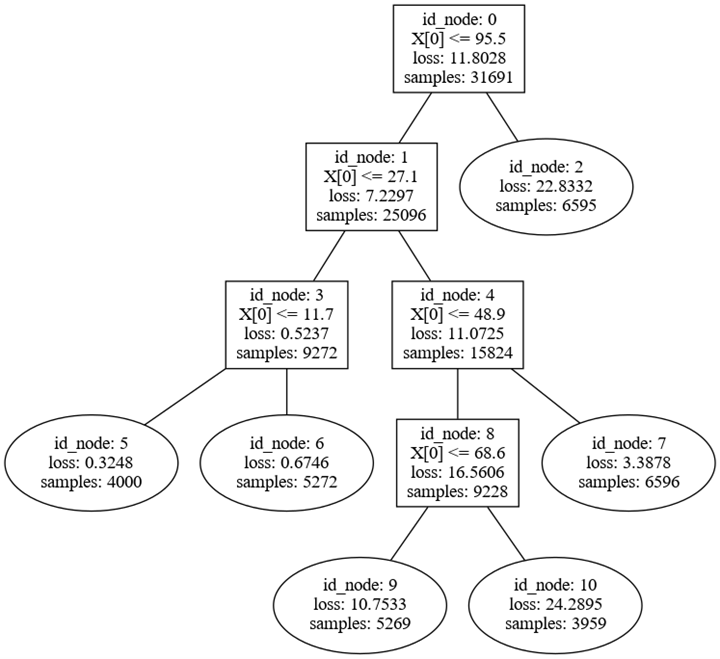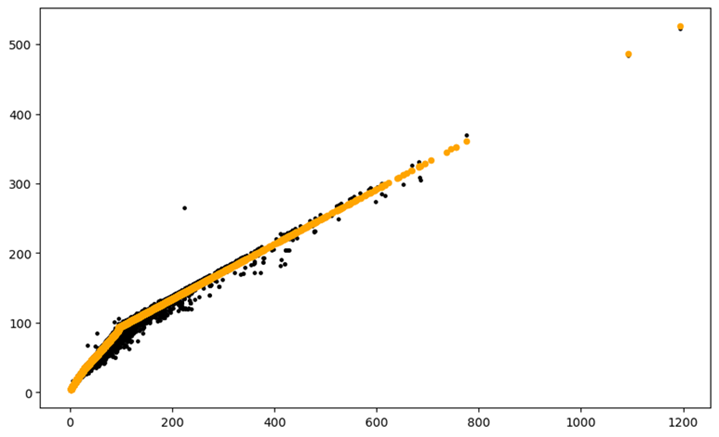## 方法四：使用Pwlf包

pwlf是一个用于实现分段线性回归的Python库。它提供了一些有用的功能，例如可视化数据和逐步添加断点以创建最佳模型。它还可以处理多元分段线性回归问题。

import pwlf

x = [1.0, 2.0, 3.0, 4.0, 5.0, 6.0, 7.0, 8.0, 9.0, 10.0]
y = [1.0, 1.5, 2.0, 4.0, 4.5, 4.0, 3.5, 3.0, 2.0, 1.0]

my_pwlf = pwlf.PiecewiseLinFit(x, y)
breaks = [3.0, 6.0]
res = my_pwlf.fit_with_breaks(breaks)
print(my_pwlf.intercepts)
print(my_pwlf.slopes)
print(my_pwlf.fit_breaks)
print(my_pwlf.ssr)


import pwlf

x = [1.0, 2.0, 3.0, 4.0, 5.0, 6.0, 7.0, 8.0, 9.0, 10.0]
y = [1.0, 1.5, 2.0, 4.0, 4.5, 4.0, 3.5, 3.0, 2.0, 1.0]

my_pwlf = pwlf.PiecewiseLinFit(x, y)
res = my_pwlf.fitfast(3) # 3表示最多可以有3个段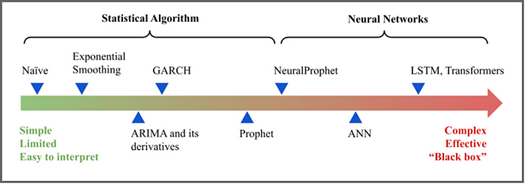##### 神经网络时间序列预测工具NeuralProphet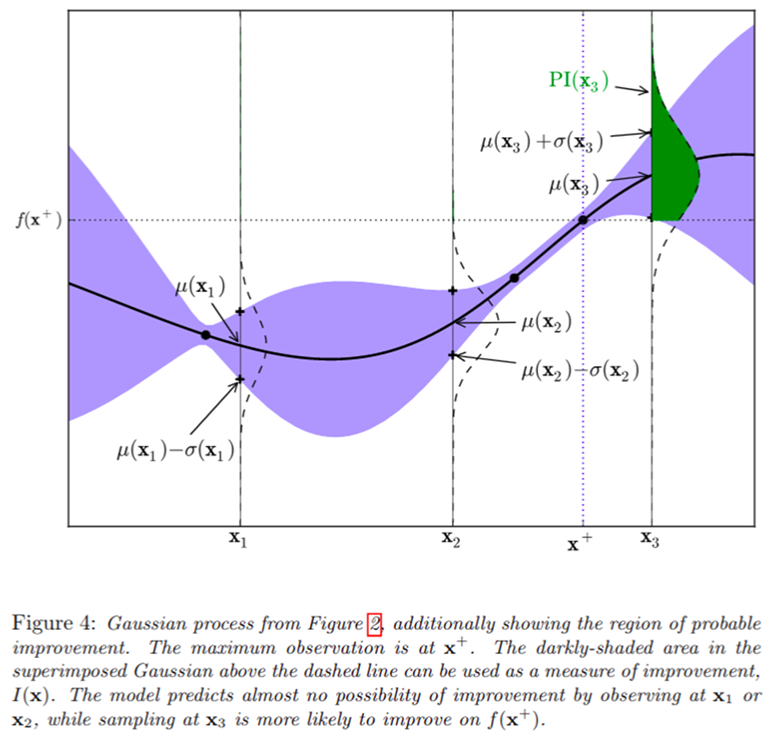##### 超参数调优之贝叶斯优化